# Statistics, Mathematical

## Statistics, Mathematical

the branch of mathematics devoted to the mathematical methods for the systematization, analysis, and use of statistical data for the drawing of scientific and practical inferences. Here, the term “statistical data” denotes information about the number of objects in some more or less general set which possess certain attributes (for example, the data in Tables la and 2a).

Field of application and method of mathematical statistics. The statistical description of a set of objects occupies an intermediate place between the individual description of each object in the set, on the one hand, and the description of the set in terms of its general properties, which does not in any way require its separation into distinct objects, on the other. Compared to the first method, statistical data are always deprived of individuality to a greater or lesser degree and have only limited value in those cases where individual data are essential (for example, a new teacher on the first day of school has only a superficial knowledge about his class obtained from some statistics presented to him by his predecessor about the number of excellent, good, satisfactory, and unsatisfactory grades). On the other hand, in comparison with data about the externally observable, summary properties of the set, statistical data make it possible to penetrate more deeply into the nature of the matter. For example, data obtained from a granulometric analysis of a rock (that is, data on the distribution of the particles forming the rock according to size) provide additional valuable information in comparison with an examination of a sample of the rock as a whole, and thus to a certain degree help elucidate the properties of the rock, the conditions of its formation, and other factors.

The method of investigation that is based on a consideration of statistical data obtained from sets of objects is termed the statistical method. The statistical method is applicable in extremely diverse fields. However, its features as applied to objects of different natures are so unique that it would be senseless to combine, for instance, socioeconomic statistics, physical statistics, and stellar statistics into a single science.

The features of the statistical method that are common to different fields include the determination of the number of objects belonging to certain groups, consideration of the distribution of quantitative attributes, application of a sampling method (in those cases where a detailed study of all the objects in an extensive set is too difficult), and use of probability theory for estimating a sufficient number of observations to reach some conclusion. This is the formal, mathematical side of statistical methods of research, which ignores the specific nature of the objects under study and constitutes the subject of mathematical statistics.

Mathematical statistics and probability theory. The connection between mathematical statistics and probability theory varies from one case to the next. Probability theory studies not just any phenomena but only random and even “probabilistically random” phenomena, that is, those for which it makes sense to speak of corresponding probability distributions. Nevertheless, probability theory also plays a definite role in the statistical study of mass phenomena of any nature, which may or may not belong to the category of probabilistically random phenomena. This is accomplished through the theory of sampling methods and the theory of measurement errors, both based on probability theory. In these cases it is not the phenomena themselves that are subject to probabilistic laws but the methods for studying them.

Probability theory plays a more important role in the statistical investigation of probabilistic phenomena. Here, the branches of mathematical statistics based on probability theory are fully applicable, such as the theory of statistical testing of probabilistic hypotheses and the theory of statistical estimation of probability distributions and associated parameters. But the area of application of these deeper statistical methods is much narrower because of the requirement that the phenomena themselves obey sufficiently definite probabilistic laws. For example, the statistical study of the behavior of turbulent streams of water or fluctuations in radio receivers is based on the theory of stationary random processes. However, the application of this same theory

Table 1a. Distribution of diameters of machine parts obtained from statistical study of mass production1
Diameter
(mm)
Basic
sample
First
sample
Second
sample
Third
sample
13.05-13.09 …11
13.10-13.14 …2
13.15-13.19 …111
13.20-13.24 …8_
13.25-13.29 …17121
13.30-13.34 …27112
13.35-13.39 …30231
13.40-13.44 …37211
13.45-13.49 …271
13.50-13.54 …2 521
13.55-13.59 …17
13.60-13.64 …71_2
13.65-13.69 …21
Total. …200101010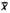. . . . .13.41613.43013.31513.385
S2. . . .2.39100.09900.14720.3602
s. . . . .0.1100.1050.1280.200
1 For explanation ofand 5 see the section in this article Simplest methods of statistical description. For explanation of s, see the section Connection between statistical distributions and probability distributions.

to the analysis of economic time series may lead to serious errors, since the assumption of the longterm presence of unchanging probability distributions that enters into the definition of a stationary process is in this case generally unacceptable.

Probabilistic laws generate corresponding statistical expressions since by virtue of the law of large numbers, probabilities are realized approximately in the form of frequencies, and mathematical expectations in the form of means.

Simplest methods of statistical description. A set of n objects may be divided according to some qualitative attribute into classes A1,A2,. . ., Ar. The statistical distribution corresponding to this division is given by indicating the numbers of objects (frequencies) n1,n2. . ., nr (whereΣ r i=i ni = n) in the individual classes. Instead of the quantities n the relative frequencies hi = ni/n are often given (these obviously satisfy the relation Σrihi = 1). If some quantitative attribute is being studied, then its distribution in a set of n objects can be given by enumerating the directly observed values of the attribute: x1, x2, . . ., xn, for example, in increasing order. However, for large n this method is cumbersome and does not clearly elucidate the essential properties of the distribution. In practice, for any large n we generally do not compile detailed tables of observed values xi but proceed from tables containing only the frequencies of classes obtained upon grouping observed values into suitable selected intervals.

Table 1b. Distribution of diameters of machine parts in basic sample1 for larger grouping intervals
DiameterNumber of parts
1 See Table la
13.00-13.2411
13.25-13.49138
13.50-13.7451
Total200

For example, the first column of Table la gives the results of measurements of the diameters of 200 machine parts, grouped according to 0.05 mm intervals. The basic sample corresponds to a normal production run. The first, second, and third samples were taken during certain time intervals as a test of the stability of the normal run. Table Ib gives the results of measurements of the machine parts in the basic sample grouped according to 0.25 mm intervals. The usual grouping into 10-20 intervals, each of which contains not more than 15-20 percent of the values xi, proves to be sufficient to note more or less all the essential properties of the distribution and to compute reliably fundamental characteristics from the class frequencies (see below). A histogram constructed from such group data graphically depicts the distribution. A histogram constructed on the basis of groupings with small intervals usually has multiple peaks and does not graphically reflect the essential properties of the distribution.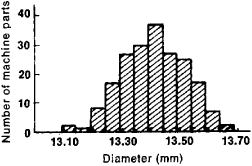Figure 1. Histogram of distribution of diameters of 200 machine parts. Length of grouping interval is 0.05 mm.

As an example, Figure 1 depicts a histogram of the distribution of the 200 diameters corresponding to the data in the first column of Table la, and Figure 2 depicts a histogram of the same distribution (the corresponding table is not provided because it is so cumbersome) with an interval of 0.01 mm. On the other hand, a grouping into excessively large intervals may lead to an unclear representation of the nature of the distribution and to gross errors in the computation of the mean and other characteristics of the distribution (see Table Ib and the corresponding histogram in Figure 3).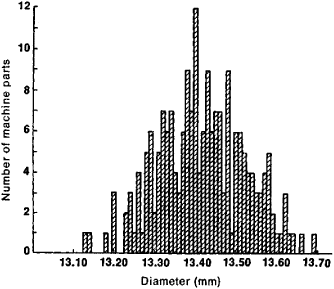Figure 2. Histogram of distribution of diameters of 200 machine parts. Length of grouping interval is 0.01 mm.

Within the limits of mathematical statistics, the question of the grouping intervals may only be regarded from a formal side: the completeness of the mathematical description of the distribution, the precision of the calculation of means from the grouped data, and so forth.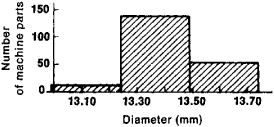Figure 3. Histogram of distribution of diameters of 200 machine parts. Length of grouping interval is 0.25 mm.

In the study of the joint distribution of two attributes, tables with two entries are used. Table 2a serves as an example of a joint distribution of two qualitative attributes. In the general case, when the set is divided into classes A1, A 2, . . . , A r according to attribute A and into classes B 1 B2, • . ., B 5 according to at-tribute B, the table consists of the numbers nij of objects simultaneously belonging to classes Ai and Bj. Summing them according to the formulas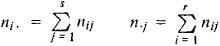we obtain the numbers in the classes Aj and Bj themselves; it is evident thatwhere n is the number of objects in the whole set. Depending on the aims of further investigation, certain relative frequencies may be calculated: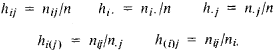Table 2a. Distribution of illness from influenza among employees of the Central Department Store in Moscow who did and did not inhale anti-influenza serum (1939)
Did not become illBecame illTotal
Did not inhale serum1,6751501,825
Inhaled serum4974501
Total2,1721542,326
Table 2b. Relative frequency of illness among employees of the Central Department Store1
Did not become illBecame illTotal
Did not inhale serum0.9180.0821,000
Inhaled serum0.9920.0081,000
1 Corresponding to data in Table 2a

For example, in investigating the effect of inhaling serum on the number of influenza cases, using the data given in Table 2a, it is natural to compute the relative frequencies, which are given in Table 2b. Table la serves as an example of a joint distribution with mixed types of attributes: the material is grouped according to one qualitative attribute (that of belonging to the basic sample generated in order to determine the mean of the production process and to the three samples generated at different times to test the stationary of this mean) and according to one quantitative attribute (the diameter of the parts).

The simplest derived characteristics of the distribution of a single quantitative attribute are the mean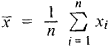and the standard deviationwhere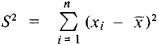In computing, S2, and D from grouped data, we use the formulas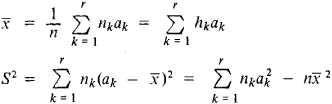or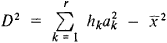where r is the number of grouping intervals and the ak are their midpoints (in the case of Table la—13.07, 13.12, 13.17, 13.22, and so forth). If the material is grouped according to excessively large intervals, then such a calculation gives inaccurate results. Sometimes in such cases it is useful to resort to special corrections for grouping. However, it makes sense to introduce these corrections only when certain probabilistic assumptions are satisfied.

Connection between statistical distributions and probability distributions; estimates of parameters; tests of probabilistic hypotheses. Presented above were only certain selected, very simple methods of statistical description, a fairly broad discipline with a well-developed system of concepts and computational procedures. The methods of statistical description are interesting not in themselves but as a means of inferring from the statistical data certain conclusions about the laws that govern the phenomena under investigation and the principles that in each case lead to the observed statistical distribution.

For example, it is natural to link the data presented in Table 2a to such a theoretical scheme. The event that a particular employee contracts influenza must be considered a random event, since the general working and living conditions of the employees examined cannot determine whether this or that employee will actually become ill but only the probability that he or she will become ill. Judging from the statistical data, the probability of contracting influenza for those who inhaled the serum (p1) and for those who did not (po) are different; the data provide a basis for the assumption that p1 is actually less than Po. The problem arises in mathematical statistics of estimating the probabilitiesp1 and po from the observed frequencies h1 =4/501 - 0.008 and/z0 = 150/1825 ~ 0.082 and testing whether the statistical evidence is sufficient to consider it established that p1 < Po (that is, that the inhalation of serum actually diminishes the probability of contracting influenza). An affirmative answer to this question for the case of the data in Table 2a is sufficiently certain even without the precise methods of mathematical statistics. But in more questionable cases it is necessary to resort to special criteria that have been worked out by mathematical statistics.

The data in the first column of Table la were gathered with the aim of establishing the precision in the manufacture of machine parts with a nominal diameter of 13.40 mm during a normal production run. The simplest assumption, which in this case may be based on certain theoretical considerations, is that the diameters of the individual machine parts may be regarded as random quantities X obeying a normal probability distributionIf this assumption is correct, then the parameters a and a2—the mean and the variance of the probability distribution—may be determined to a sufficient accuracy by the corresponding characteristics of the statistical distribution (since the number of observations n = 200 is sufficiently great). As an estimate of the theoretical variance a2 it is preferable to take the unbiased estimate

s2 = s2/(n – 1)

rather than the sample variance D2 = S2 /n.

There exists no general (that is, applicable to any probability distribution) unbiased estimate for the theoretical standard deviation. As an estimate (strictly speaking, a biased one) for cr, we often use s. The accuracy of the estimates x and s for a and cr is shown by their respective variances, which in the case of a normal distribution (1) have the form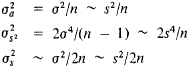where the sign ~ denotes approximate equality for large n. In this manner, having agreed to append to the estimates their variance with the sign ±, we have for large n under the assumption of a normal distribution (1)For the data in the first column of Table la, the formulas (2) give

σ = 13.416 ± 0.008

σ = 0.110 ± 0.006

The sample size n = 200 is sufficient to ensure the validity of using these formulas based on the theory of large samples.

Table 3. Dependence of α and ω = 1 - α on k
k1.962.583.003.29
α0.0500.0100.0030.001
ω0.0950.9900.9970.999

The use of the formulas of the theory of large samples, which have been established only as limiting formulas for n→, can serve only as a first approximation in the examination of the data in the subsequent columns of Table la, each of which is compiled on the basis of ten measurements. As before, the values x and s can be used as approximate estimates for the parameters a and or; however, in order to determine the accuracy and reliability of these estimates it is necessary to apply the theory of small samples. In comparing according to the rules of mathematical statistics the values x and s appearing in the last lines of Table la for the three samples with the normal values a and σ, as estimated by the first column of the table, we can draw the following conclusions: the first sample does not provide a basis for assuming a substantial change in the characteristics of the production process, the second sample provides a basis for concluding that the mean diameter a has decreased, and the third sample provides a basis for concluding that the variance has increased.

All rules for statistically estimating parameters and testing hypotheses, which are based on probability theory, operate only at some definite significance level ω < 1, that is, they may lead to erroneous results with a probability α = 1 ω. For example, if, under the assumption of a normal distribution and a known theoretical variance σ2, α is estimated using xē by stating that a satisfies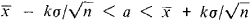then the probability of error equals a, which is connected to kby the relation (see Table 3):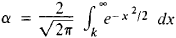The question concerning a reasonable choice of significance level under given specific conditions (for example, in working out rules for the statistical control of mass production) is very important. In this case, the desire to apply only rules that have a high (close to unity) level of significance is opposed by the circumstance that when there is a limited number of observations such rules allow only very poor conclusions (for example, they do not make it possible to establish an inequality of probabilities even when there is a marked inequality in the frequencies).

Sampling method. In the preceding analysis, the results of observations that were to be used to estimate the probability distribution or its parameters were assumed (although this was not mentioned) to be independent. A well-studied example of the use of dependent observations is the estimation of the statistical distribution or its parameters in a “population” of N objects by means of a “sample” drawn from it containing n < N objects.

Terminological Note. Often, a set of n observations made for the purpose of estimating a probability distribution is also called a sample. This explains, for instance, the origin of the term “theory of small samples” used above. This terminology is connected with the fact that the probability distribution is often considered as a statistical distribution of a hypothetical infinite population, and it is conventionally considered that the n observed objects have been “selected” from this population. These ideas do not have clear meaning. In the proper sense of the word, sampling always presupposes an initial finite general set.

The following may serve as an example of the application of the sampling method. In a batch of N articles, let L be the number of defective ones; n < N objects are randomly selected (for example, n = 100 for N = 10,000) from the batch. The probability that the number l of defective articles in the sample is equal to m isThus, l and the corresponding relative frequency h = l/n prove to to be random variables whose distribution depends on the parameter L or, which is the same thing, on the parameter H = L/N. The problem of estimating the relative frequency Hfrom the sampled relative frequency h is similar to the problem of estimating the probability p from the relative frequency h in n independent trials. For large n with a probability close to unity, the approximate equality p ~ h holds for the problem of estimating the probability and the approximate equality H ~ h holds for the problem of estimating the relative frequency. However, for the problem of estimating H, the formulas are more complex and the deviation of h from H on the average is somewhat smaller than the deviation of h from p in the problem of estimating probability (for the same n). Thus, the estimate of the fraction H of defective articles in the batch given by the fraction h of defective articles in the sample for a given sample size n is always (for any N) somewhat more accurate than the estimate of the probability p given by the relative frequency h in independent trials. When N/n → ∞, the formulas for the sampling problem asymptotically approach the formulas for the problem of estimating the probability p.

Additional problems of mathematical statistics. The aforementioned methods of estimating parameters and testing hypotheses are based on the assumption that the number of observations necessary to attain a given accuracy in the conclusions has been determined in advance (before the trials). However, the a priori determination of the number of observations is often not expedient since by determining the number of trials during the course of the experiment rather than by fixing it in advance, we may decrease its mathematical expectation. This circumstance was first observed in the case involving the choice of one of two hypotheses by means of a sequence of independent trials. The appropriate procedure, first proposed in connection with problems of statistical quality control, consists in the following: from the results of the already conducted observations, at each step a decision is made (1) to carry out the next trial, or (2) to discontinue the trials and accept the first hypothesis, or (3) to discontinue the trials and accept the second hypothesis. By a suitable choice of quantitative characteristics, in such a procedure it is possible (with the same accuracy in the conclusions) on the average to cut in half the number of observations needed in contrast to a procedure with a sample of fixed size.

The development of the methods of sequential analysis has led to the study of controlled random processes, on the one hand, and to the emergence of a general theory of statistical decisions, on the other. The latter theory proceeds from the fact that the results of sequentially conducted observations serve as the basis for making certain decisions (intermediate ones—to continue the trials or not—and final ones—made on discontinuing the trials). In problems involving estimation of parameters, the results of the final decision are numbers (the values of the estimates), while in those involving testing hypotheses they are the accepted hypotheses. The goal of the theory is to indicate the rules for making decisions that minimize the average risk or loss (the risk depends on the probability distributions of the results of the observations, on the final decision made, on the cost of conducting the trials, and on other factors).

Questions concerning the expedient allocation of effort in conducting a statistical analysis of a phenomenon are considered in the theory of experimental design, which has become an important part of modern mathematical statistics.

The development and refinement of the general concepts of mathematical statistics have been accompanied by the development of its individual branches, such as analysis of variance, statistical analysis of random processes, and multivariate statistical analysis. New analyses have emerged in the field of regression analysis. The so-called Bayes approach plays a large role in problems of mathematical statistics.

History. The first principles of mathematical statistics appear in the works of the creators of the theory of probability—Jakob Bernoulli (late 17th century and early 18th), P. de Laplace (second half of the 18th century and early 19th), and S. Poisson (first half of the 19th century). In Russia, methods of mathematical statistics for use in demography and insurance were developed by V. Ia. Buniakovskii (1846) on the basis of probability theory. The work of the Russian classical school of probability theory in the second half of the 19th century and in the early 20th (P. L. Chebyshev, A. A. Markov, A. M. Liapunov, S. N. Bernshtein) was of decisive significance for the future development of mathematical statistics. Many questions in the theory of statistical estimations were essentially worked out on the basis of the theory of errors and the method of least squares by K. Gauss (first half of the 19th century) and A. A. Markov (end of the 19th century and beginning of the 20th). The work of L. A. Quetelet (19th century, Belgium), F. Gallon (19th century, Great Britain), and K. Pearson (end of the 19th century and beginning of the 20th, Great Britain) was of great importance, but, in terms of the degree of use of probability theory, it lagged behind the Russian school. Pearson worked extensively on the compilation of tables of functions necessary for the application of the methods of mathematical statistics.

The concepts of the Anglo-American school [Student (pseudonym of W. Gosset), R. Fisher, and E. Pearson of Great Britain and J. Neyman and A. Wald of the USA], which arose in the 1920’s, played an extremely important role in the establishment of the theory of small samples, the general theory of

Table 1. Stubble plows produced in the USSR
Disc stubble plowsShare stubble plows
LD-20LD-15LD-10LD-5PL-10-25LN-5-258PL-5-25PLS-5-25A
Number of sections or frames. . . . .16128410555
Operating width (m) . . . . .20151052.51.251.251.25
Angle of attack (degrees) . . . . .20-353515-3515-35
Productivity (hectares per hour) . . . . .187.66.542110.5

statistical estimation and hypothesis testing (freed from assumptions about the presence of a priori distributions), and sequential analysis. In the USSR important results in mathematical statistics were obtained by V. I. Romanovskii; E. E. Slutskii, the author of important works on the statistics of dependent stationary sequences; N. V. Smirnov, who laid the foundations of the theory of nonparametric methods in mathematical statistics; and Iu. V. Linnik, who enriched the analytic apparatus of mathematical statistics with new methods. Mathematical statistics has been the basis for particularly intensive development of statistical methods for the study and control of mass production and statistical methods used in physics, hydrology, climatology, stellar astronomy, biology, medicine, and other fields.

Among the journals that publish works on mathematical statistics are Annals of Statistics (known until 1973 as Annals of Mathematical Statistics), International Statistical Institute Review, Biometrika, and Journal of the Royal Statistical Society. There are scientific associations that support research in mathematical statistics and applications. An important role is played by the International Statistical Institute (ISI), centered in Amsterdam, as well as by the International Association for Statistics in Physical Sciences (IASPS), which was founded by the ISI.

### REFERENCES

Cramér, H. Matematicheskie melody statistiki. Moscow, 1948. (Translated from English.)
Van der Waerden, B. L. Matematicheskaia statistika. Moscow, 1960. (Translated from German.)
Smirnov, N. V., and I. V. Dunin-Barkovskii. Kurs teorii veroiatnostei i matematicheskoi statistiki dlia tekhnicheskikh prilozhenii, 3rd ed. Moscow, 1969.
Bol’shev, L. N., and N. V. Smirnov. Tablitsy matematicheskoi statistiki. Moscow, 1968.
Linnik, lu. V. Metod naimen ’shikh kvadratov …, 2nd ed. Moscow, 1962.
Hald, A. Matematicheskaia statistika s tekhnicheskimi prilozheniiami. Moscow, 1956. (Translated from English.)
Anderson, T. Vvedenie v mnogomernyi statisticheskii analiz. Moscow, 1963. (Translated from English.)
Kendall, M. G., and A. Stuart. Teoriia raspredelenii. Moscow, 1966. (Translated from English.)

A. N. KOLMOGOROV and IU. V. PROKHOROV

References in periodicals archive ?
The programme includes 17 courses in the fields of biology, physics, chemistry, cognitive science, cyber security, mathematics, nanoscience, statistics, mathematical information technology and information systems science.
For his work, Colchero used statistics, mathematical derivations and computer simulations with data from wild populations of 24 vertebrate species.
The chapters/headings are: numbers, geometry, algebra, discrete mathematics, analysis, logic, metamathematics, probability and statistics, mathematical physics, games and recreation.

Site: Follow: Share:
Open / Close